#### Piecewise-smooth vector fields

It will be important to allow vector fields that are smooth only over a finite number of patches. At a switching boundary between two patches, a discontinuous jump may occur. For example, suppose that an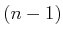-dimensional switching boundary,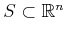, is defined as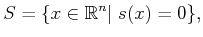(8.17)

in which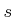is a function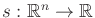. If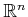has dimensionandis not singular, then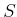has dimension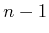. Define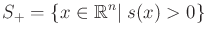(8.18)

and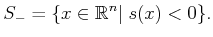(8.19)

The definitions are similar to the construction of implicit models using geometric primitives in Section 3.1.2. Suppose that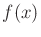is smooth over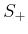andbut experiences a discontinuous jump at. Such differential equations model hybrid systems in control theory [137,409,634]. The task there is to design a hybrid control system. Can we still determine a solution trajectory in this case? Under special conditions, we can obtain what is called a solution to the differential equations in the sense of Filipov [338,846].

Let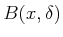denote an open ball of radius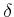centered at. Let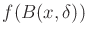denote the set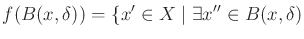for which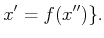(8.20)

Let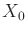denote any subset ofthat has measure zero (i.e.,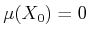). Let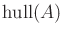denote the convex hull of a set,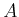, of points in. A path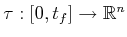is called a solution in the sense of Filipov if for almost all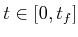,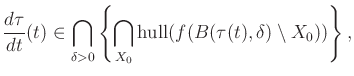(8.21)

in which the intersections are taken over all possible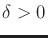and sets,, of measure zero. The expression (8.21) is actually called a differential inclusion  because a set of choices is possible for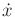. The for almost all'' requirement means that the condition can even fail to hold on a set of measure zero in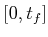. Intuitively, it says that almost all of the velocity vectors produced by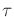must point between'' the velocity vectors given by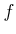in the vicinity of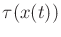. The between'' part comes from using the convex hull. Filipov's sense of solution is an incredible generalization of the solution concept in the sense of Caratheodory. In that case, every velocity vector produced bymust agree with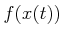, as given in (8.14). The condition in (8.21) allows all sorts of sloppiness to appear in the solution, even permittingto be discontinuous.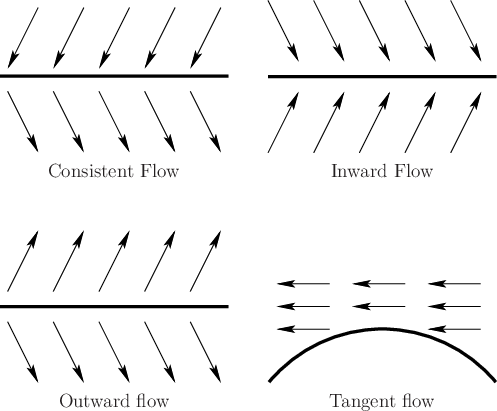Many bizarre vector fields can yield solutions in the sense of Filipov. The switching boundary model is relatively simple among those permitted by Filipov's condition. Figure 8.7 shows various cases that can occur at the switching boundary. For the case of consistent flow, solutions occur as you may intuitively expect. Filipov's condition, (8.21), requires that atthe velocity vector ofpoints between vectors before and after crossing(for example, it can point down, which is the average of the two directions). The magnitude must also be between the two magnitudes. For the inward flow case, the integral curve moves along, assuming the vectors inside ofpoint in the same direction (within the convex hull) as the vectors on either side of the boundary. In applications that involve physical systems, this may lead to oscillations around. This can be alleviated by regularization, which thickens the boundary  (the subject of sliding-mode control addresses this issue ). The outward flow case can lead to nonuniqueness if the initial state lies in. However, trajectories that start outside ofwill not cross, and there will be no such troubles. If the flow is tangent on both sides of a boundary, then other forms of nonuniqueness may occur. The tangent-flow case will be avoided in this chapter.

Steven M LaValle 2020-08-14